# random header | Set 2 (Distributions)

• Difficulty Level : Easy
• Last Updated : 29 May, 2017

Set 1 (Generators)

Distributions

Want to learn from the best curated videos and practice problems, check out the C++ Foundation Course for Basic to Advanced C++ and C++ STL Course for foundation plus STL.  To complete your preparation from learning a language to DS Algo and many more,  please refer Complete Interview Preparation Course.
1. uniform_int_distribution: It produces random integer values i, which are uniformly distributed on the closed interval [a,b], which is described by the following probability mass function: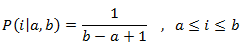• operator(): It generates the random number that are distributed according to the probability function.
• min: It returns the greatest lower bound of the range of values returned by operator(), which is the distribution parameter ‘a’ for uniform_int_distribution.
• max: It returns the least upper bound of the range of values returned by operator(), which is the distribution parameter ‘b’ for uniform_int_distribution.
• reset: It resets the distribution, so that on the subsequent uses the result does not depends on the values already produced by it.
 `// C++ program to illustrate``// the use of operator()``// in uniform_int_distribution``#include ``#include ``using` `namespace` `std;`` ` `// Driver program``int` `main()``{``     ` `    ``// Constructing a trivial random generator engine ``    ``unsigned s = 2;``     ` `    ``// The random number generator``    ``default_random_engine generator (s);``     ` `    ``uniform_int_distribution<``int``> distribution(1,10);``    ``cout << ``"Some random numbers between 1 and 10"``;``    ``for` `(``int` `i = 0; i < 10; ++i)``        ``cout << distribution(generator) ;``     ` `    ``cout << endl;``     ` `    ``return` `0;``}`

Output:

```Some random numbers between 1 and 10: 1 3 6 10 1 5 1 4 4 9
```
 `// C++ program to illustrate``// the use of reset``// in uniform_int_distribution``#include ``#include ``using` `namespace` `std;`` ` `//Driver program``int` `main()``{``         ` `    ``//the random number generator``    ``default_random_engine generator;``     ` `    ``// Initialising the uniform distribution``    ``uniform_int_distribution<``int``> distribution(1, 1000);``     ` `    ``// First random number is generated``    ``cout << distribution(generator) << endl;``     ` `    ``//Resets the distribution``    ``distribution.reset();``     ` `    ``// Second random number is ``    ``//generated independent of previous number``    ``cout << distribution(generator) << endl;``     ` `    ``return` `0;``}`

Output:

```1
132
```
2. uniform_real_distribution: It is the random number distribution that produces floating-point values , which is described by the following probability density function: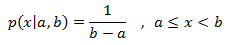• operator(): It returns a new random number that follows the distribution’s parameters.
• min: It returns the greatest lower bound of the range of values returned by operator(), which is the distribution parameter ‘a’ for uniform_real_distribution.
• max: It returns the least upper bound of the range of values returned by operator(), which is the distribution parameter ‘b’ for uniform_real_distribution.
• reset: It resets the distribution, so that on the subsequent uses the result does not depend on values already produced by it.
 `// C++ program to illustrate``// the use of operator()``// in uniform_int_distribution``#include ``#include ``using` `namespace` `std;`` ` `// Driver program``int` `main()``{``     ` `    ``// Constructing a trivial random generator engine ``    ``unsigned s = 2;``     ` `    ``// The random number generator``    ``default_random_engine generator (s);``     ` `    ``uniform_int_distribution<``int``> distribution(1,10);``    ``cout << ``"Random numbers between 1 and 10"``;``    ``for` `(``int` `i = 0; i< 10; ++i)``        ``cout << distribution(generator) ;``     ` `    ``cout << endl;``     ` `    ``return` `0;``}`

Output:

```some random numbers between 0.0 and 10.0:
0.150031
9.77072
3.36669
7.06447
5.11455
8.43061
1.93792
7.78965
8.31532
5.14354
```
 `// C++ program to illustrate``// the use of reset``// in uniform_real_distribution``#include ``#include ``using` `namespace` `std;`` ` `// Driver program``int` `main()``{``    ``default_random_engine generator;``    ``uniform_real_distribution<``double``> distribution(0.0,100.0);``     ` `    ``// It prints two independent values:``    ``// First random number is generated``    ``cout << distribution(generator) << endl;``     ` `    ``//Resets the distribution``    ``distribution.reset();``     ` `    ``// Second random number is ``    ``//generated independent of previous number``    ``cout << distribution(generator) << endl;``     ` `    ``return` `0;``}`

Output:

```13.1538
45.865
```

II. Related to bernoulli trials: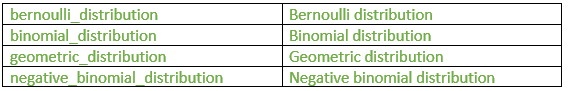1. bernoulli_distribution: It is the random number distribution that produces bool values according to a Bernoulli distribution, given by the following probability mass function:• operator(): It returns a new random number.
• min: It returns the greatest lower bound of the range of values returned by operator(), which for bernoulli_distribution is false.
• max: It returns the least upper bound of the range of values returned by operator(), which for bernoulli_distribution is true.
 `// C++ program to illustrate``// the bernoulli_distribution``#include ``#include ``using` `namespace` `std;`` ` `//Driver program``int` `main()``{``    ``const` `int` `temp=500;``     ` `    ``//The random number generator``    ``default_random_engine generator;``     ` `    ``//Initialising the bernoulli distribution``    ``bernoulli_distribution distribution(0.7);``     ` `    ``// count number of trues``    ``int` `count=0; ``     ` `    ``for` `(``int` `i = 0; i < temp; ++i) ``    ``{``         ` `        ``// checking for true condition ``        ``if` `(distribution(generator)) ``        ``count++;``    ``}``     ` `    ``cout << ``"bernoulli_distribution (0.7) x 500:"` `<< endl;``    ``cout << ``"true: "` `<< count << endl;``    ``cout << ``"false: "` `<< temp-count << endl;``     ` `    ``return` `0;``}`

Output:

```bernoulli_distribution (0.7) x 500:
true:  360
false: 140
```
 `// C++ program to``// illustrate the use of reset``#include ``#include ``using` `namespace` `std;`` ` `//Driver program``int` `main()``{``    ``// Random number generator``    ``default_random_engine generator;``     ` `    ``// Initialising the bernoulli distribution``    ``bernoulli_distribution distribution;``     ` `    ``// print two independent values:``    ``cout << distribution(generator) << endl;``     ` `    ``// use of reset``    ``// Generates second output without``    ``// the effect of first output ``    ``distribution.reset();``    ``cout << distribution(generator) << endl;`` ` `return` `0;``}`

Output:

```1
1
```
2. binomial_distribution: It is the random number distribution that produces integers according to a binomial discrete distribution, which is given by this probability mass function:• operator(): It generates a new random number.
• max: It returns the least upper bound of the range given by operator(), which for binomial_distribution is the distribution parameter t.
• min: It returns the greatest lower bound of the range given by member operator(), which for binomial_distribution is always zero.
• reset: It resets the distribution, so that subsequent uses of the object do not depend on values already produced by it.
 `// C++ program to illustrate``// the use of binomial_distribution``#include ``#include ``#include ``using` `namespace` `std;`` ` `int` `main()``{``     ` `    ``// construct a trivial random ``    ``//generator engine from a time-based seed:``    ``unsigned seed = chrono::system_clock::now().time_since_epoch().count();``    ``default_random_engine generator (seed);``     ` `    ``// Initialising binomial distribution``    ``binomial_distribution<``int``> distribution (15, 0.4);``     ` `    ``cout << ``"some binomial results (t=15, p=0.4): "``;``    ``for` `(``int` `i = 0; i < 15; ++i)``    ``{``             ` `        ``// Use of operator()``        ``cout << distribution(generator) << ``" "``;``    ``}``    ``cout << endl;``     ` `    ``return` `0;``}`

Output:

```some binomial results (t=15, p=0.4): 7 6 7 8 4 6 7 6 9 3 5 6 4 6 7
```
 `// C++ program to illustrate``// the use of binomial_distribution``#include ``#include ``#include ``using` `namespace` `std;`` ` `int` `main()``{``     ` `    ``// construct a trivial random ``    ``//generator engine from a time-based seed:``    ``unsigned seed = chrono::system_clock::now().time_since_epoch().count();``    ``default_random_engine generator (seed);``     ` `    ``// Initialising binomial distribution``    ``binomial_distribution<``int``> distribution (15, 0.4);``     ` `    ``cout << ``"some binomial results (t=15, p=0.4): "``;``    ``for` `(``int` `i = 0; i < 15; ++i)``    ``{``             ` `        ``// Use of operator()``        ``cout << distribution(generator) << ``" "``;``    ``}``    ``cout << endl;``     ` `    ``return` `0;``}`

Output:

```57
52
```
3. geometric_distribution: It is a random number distribution that produces integers according to a geometric discrete distribution, given by the following probability mass function:• operator(): It returns a new random number that follows the distribution’s parameters.
• max: It returns least upper bound of the range given by operator().
• min: It returns the minimum value given by operator().
• reset: It resets the distribution, so that subsequent uses of the object do not depend on values already produced by it.
 `// C++ program to illustrate``//the use of geometric_distribution ``#include ``#include ``#include ``#include ``using` `namespace` `std;`` ` `int` `main()``{``    ``// construct a trivial random``    ``// generator engine from a time-based seed:``    ``int` `seed = chrono::system_clock::now().time_since_epoch().count();``    ``default_random_engine generator (seed);``     ` `    ``// Initialises the geometric distribution``    ``geometric_distribution<``int``> distribution (1.0 / 5);``     ` `    ``cout << ``"Plus sign is 5 spaces away from the next :"` `<< endl;``    ``for` `(``int` `i = 0; i < 10 ; ++i) ``    ``{``        ``int` `number = distribution(generator);``        ``cout << string (number,``' '``) << ``"+"``;``    ``}`` ` `return` `0;``}`

Output:

```each plus sign is 5 spaces away from the next :
++ + +   +  ++     +        ++
```
 `// C++ program to illustrate ``// the use of reset``#include ``#include ``using` `namespace` `std;`` ` `// Driver program``int` `main()``{``     ` `    ``// Random number generator ``    ``default_random_engine generator;``     ` `    ``// Initialising the geometric distribution``    ``geometric_distribution<``int``> distribution(0.3);``     ` `    ``// Prints two independent values:``    ``// Generates the first value``    ``cout << distribution(generator) << endl;``     ` `    ``// Use of reset``    ``distribution.reset();``     ` `    ``// Generates second value``    ``cout << distribution(generator) << endl;``     ` `    ``return` `0;``}`

Output:

```0
1
```
4. negative_binomial_distribution: It is a random number distribution that produces integers according to a negative binomial discrete distribution (also known as Pascal distribution), given by the following probability mass function: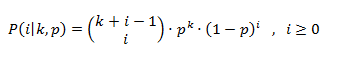• operator():It returns a new random number which follows the distribution’s parameters.
• max:It returns least upper bound of the range given by operator().
• min:It returns the minimum value given by operator(),which for negative_binomial_distribution is always zero.
• reset: It resets the distribution, so that subsequent uses of the object do not depend on values already produced by it.
 `// C++ program to illustrate``// the use of operator() in``// negative_binomial_distribution ``#include ``#include ``#include ``using` `namespace` `std;`` ` `// Driver program``int` `main()``{``    ``// construct a trivial random ``    ``// generator engine from a time-based seed:``    ``unsigned seed = chrono::system_clock::now().time_since_epoch().count();``    ``default_random_engine generator (seed);``     ` `    ``// Initialising negative binomial distribution``    ``negative_binomial_distribution<``int``> distribution (6,0.7);``     ` `    ``cout << ``"Negative binomial results (t=6, p=0.7): "``;``    ``for` `(``int` `i = 0; i < 15; ++i)``        ``{``            ``// Use of operator``            ``cout << distribution(generator) << ``" "``;``        ``}``     ` `    ``cout << endl;``     ` `    ``return` `0;``}`

Output:

```Negative binomial results (t=6, p=0.7): 2 6 3 1 4 1 4 1 2 0 7 3 4 4 4
```
 `// C++ program to illustrate``// the use of reset in``// negative_binomial_distribution::``#include ``#include ``using` `namespace` `std;`` ` `// Driver program``int` `main()``{``     ` `    ``// Random number generator ``    ``default_random_engine generator;``     ` `    ``// Initialising the negative binomial distribution``    ``negative_binomial_distribution<``int``> distribution(20, 0.5);``     ` `    ``// print two independent values:``    ``// Generates the first value``    ``cout << distribution(generator) << endl;``     ` `    ``// Use of reset``    ``distribution.reset();``     ` `    ``// Generates the second value``    ``cout << distribution(generator) << endl;``     ` `    ``return` `0;``}`

Output:

```23
30
```

III.Piece wise distributions:1. discrete_distribution: It is a random number distribution that produces integer values according to a discrete distribution.
• operator(): It returns a new random number that follows the distribution’s parameters.
• max: It returns the least upper bound of the range given by operator().
• min: It returns the greatest lower bound of the range given by operator().
• reset: It resets the distribution, so that subsequent uses of the object do not depend on values already produced by it.
 `// C++ program to illustrate the``// use of operator() in``// discrete_distribution``#include ``#include ``using` `namespace` `std;`` ` `int` `main()``{``     ` `    ``// number of experiments``    ``int` `n = 10000; ``     ` `    ``// maximum number of stars to distribute``    ``int` `m = 100; ``     ` `    ``// Random number generator``    ``default_random_engine generator;``     ` `    ``//Initialising discrete distribution``    ``discrete_distribution<``int``> distribution { 2, 2, 1, 1, 2, 2, 1, 1, 2, 2 };``     ` `    ``int` `p = {};``     ` `    ``// use of operator()``    ``for` `(``int` `i = 0; i < n; i++) ``    ``{``        ``int` `number = distribution(generator);``        ``p[number]++;``    ``}``     ` `    ``cout << ``"a discrete_distribution:"` `<< endl;``    ``for` `(``int` `i = 0; i < 10; ++i)``    ``{``        ``cout << i << ``": "` `<< string(p[i]*m/n,``'*'``) << endl;``    ``}``     ` `    ``return` `0;``}`

Output:

```a discrete_distribution:
0: ************
1: *************
2: *****
3: ******
4: ************
5: ************
6: ******
7: ******
8: ************
9: ************
```
 `// C++ program to illustrate``//the use of reset in``//discrete_distribution``#include ``#include ``using` `namespace` `std;`` ` `// Driver program``int` `main()``{``     ` `    ``// Random number generator ``    ``default_random_engine generator;``     ` `    ``// Initialising the discrete distribution``    ``discrete_distribution<``int``> distribution {20,20,30,40};``     ` `    ``// print two independent values:``    ``// Generates the first value``    ``cout << distribution(generator) << endl;``     ` `    ``// Use of reset``    ``distribution.reset();``     ` `    ``// Generates the secong value``    ``cout << distribution(generator) << endl;``     ` `    ``return` `0;``}`

Output:

```0
2
```
2. piecewise_constant_distribution: It is a random number distribution that produces floating-point values that are uniformly distributed over each of a sequence of contiguous subintervals, given by following probability density function: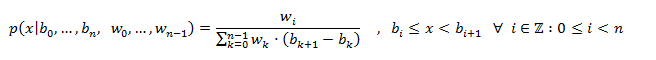• operator(): It returns a new random number that follows the distribution’s parameters.
• max: It returns the least upper bound of the range given by operator().
• min: It returns the greatest lower bound of the range given by operator().
• reset: It resets the distribution, so that subsequent uses of the object do not depend on values already produced by it.
 `// C++ program to illustrate the``// use of reset in``// piecewise_constant_distribution``#include ``#include ``using` `namespace` `std;`` ` `// Driver program``int` `main()``{``     ` `    ``// Random number generator``    ``default_random_engine generator;``     ` `    ``// Initialisind piecewise_constant_distribution``    ``piecewise_constant_distribution<``double``> distribution ``                ``( 4, 0.0, 10.0, [](``double` `x){``return` `x;} );``     ` `    ``// print two independent values:``    ``// Generates the first value``    ``// Use of operator()``    ``cout << distribution(generator) << endl;``     ` `    ``// Use of reset``    ``distribution.reset();``     ` `    ``// Generates second value``    ``cout << distribution(generator) << endl;``     ` `    ``return` `0;``}`

Output:

```3.4205
6.6692
```
3. piecewise_linear_distribution: It is a random number distribution that produces floating-point values that are distributed over a sequence of contiguous subintervals.
• operator():It returns a new random number that follows the distribution’s parameters.
• max: It returns the least upper bound of the range given by operator().
• min: It returns the greatest lower bound of the range given by operator().
• reset: It resets the distribution, so that subsequent uses of the object do not depend on values already produced by it.
 `// C++ program to illustrate the``// use of reset in``// piecewise_linear_distribution``#include ``#include ``using` `namespace` `std;`` ` `// Driver program``int` `main()``{``     ` `    ``// Random number generator``    ``default_random_engine generator;``     ` `    ``// Initialising piecewise_linear_distribution``    ``piecewise_linear_distribution<``double``>``        ``distribution ( 5, 0.0, 10.0, [](``double` `x){``return` `x+1.0;} );``     ` `    ``// print two independent values:``    ``// generates first value``    ``// use of operator()``    ``cout << distribution(generator) << endl;``     ` `    ``// Use of reset``    ``distribution.reset();``     ` `    ``// generates second value``    ``cout << distribution(generator) << endl;``     ` `    ``return` `0;``}`

Output:

```2.48143
6.07656
```

This article is contributed by Shambhavi Singh. If you like GeeksforGeeks and would like to contribute, you can also write an article using contribute.geeksforgeeks.org or mail your article to contribute@geeksforgeeks.org. See your article appearing on the GeeksforGeeks main page and help other Geeks.